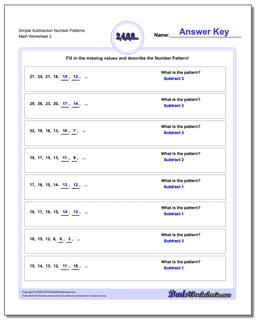One Dad. Four daughters. 9,251 worksheets... and counting!# Simple Subtraction Number Patterns (Second Worksheet)

PropertyValue
DescriptionSimple Subtraction Number Patterns: Number pattern problems using only subtraction operations. (Second Worksheet)
Resource TypeWorksheet